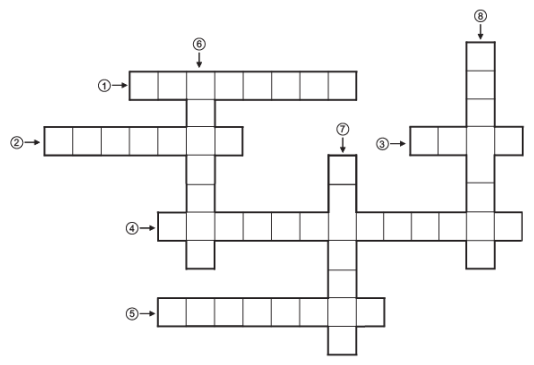Courses

# Formative Assessment- Understanding Quadrilaterals Class 8 Notes | EduRev

## Mathematics (Maths) Class 8

Created by: Indu Gupta

## Class 8 : Formative Assessment- Understanding Quadrilaterals Class 8 Notes | EduRev

The document Formative Assessment- Understanding Quadrilaterals Class 8 Notes | EduRev is a part of the Class 8 Course Mathematics (Maths) Class 8.
All you need of Class 8 at this link: Class 8

Question 1: Complete the following crossword puzzle using the given directions for Across [from left to right] and Down [from top to bottom].Directions:
Accross: (1) The points where, the sides of a polygon meet are called __________.
(2) A polygon made by six sides is called a __________.
(3) The side joining two vertices is called a __________.
(4) A four sided polygon is called a __________.
(5) A five sided polygon is called a __________.

Down:
(6) A parallelogram having all of its four sides equal is called a __________.
(7) A simple closed figure made of only line-segments is called a __________.
(8) The line segment joining the opposite sides of a polygon (except-triangle) is called a __________ of the polygon.

Question 2: Match the following:

 Column A Column B (a) The parallelogram that is inscribed in a circle is a ____ (i) Square (b) The parallelogram having all of its sides equal is called a_______ (ii) Rectangle (c) The diagonals of a quadrilateral areunequal and bisect each other necessarily at right angles. It is a _____ (iii) Kite (d) The diagonals of a parallelogram areequal and bisect each other at rightangles. It is a ______ (iv) Rhombus

Question 3: Choose the appropriate answer:
(i) Which of the following is a regular quadrilateral? (a) a rhombus (b) a square (c) a rectangle (d) a parallelogram
(ii) Which of the following is not a parallelogram?
(a) square (b) Trapezium (c) Rhombus (d) Rectangle
(iii) Which of the following quadrilateral is having every internal angle equal to 90 °
(a) Rhombus (b) parallelogram (c) kite (d) Rectangle
(iv) Which of the following is the sum of an exterior angle and its adjacent interior angle?
(a) A straight angle (b) A right angle (c) A complete angle

Question 4: Answer the following questions:
(i) For a regular polygon of  n sides (n—2) × 180° is the measure of the sum of its interior angles or exterior angles?
(ii) If the sum of all the exterior angles of a quadrilateral is 360° then what is the sum of all the exterior angles of an octagon?
(iii) Tetragon is the other name of which polygon–a quadrilateral or a triangle?
(iv) What do we call a trapezium having its nonparallel sides equal?

1. (1) → VERTICES
(2) → HEXAGON
(3) → EDGE

(5) → PENTAGON
(6) → RHOMBUS
(7) → POLYGON
(8) → DIAGONAL

2. (a) → (ii) (b) → (iv) (c) → (iii) (d) → (i)
3. (i) (b) (ii) (b) (iii) (d) (iv) (a)
4. (i) Sum of interior angles
(ii) 360°
(iv) An isosceles trapezium

Offer running on EduRev: Apply code STAYHOME200 to get INR 200 off on our premium plan EduRev Infinity!

,

,

,

,

,

,

,

,

,

,

,

,

,

,

,

,

,

,

,

,

,

;